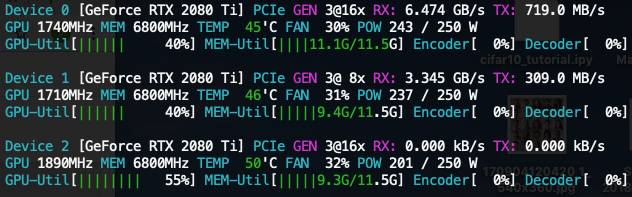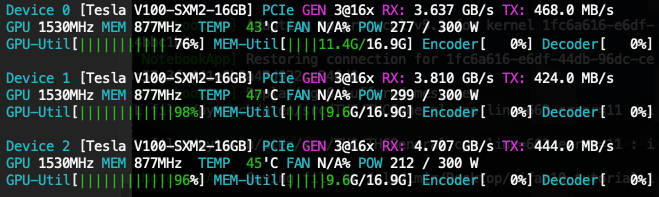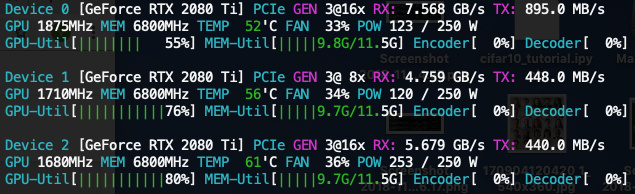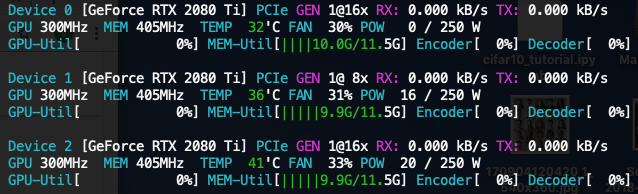# Lesser memory consumption with a larger batch in multi GPU setup

(Vladimir Iashin) #1

-Minimal- working example
``````import torch
import torchvision
import torchvision.transforms as transforms
import torch.nn as nn
import torch.nn.functional as F
import torch.optim as optim

B = 4400
# B = 4300
transform = transforms.Compose(
[transforms.ToTensor(),
transforms.Normalize((0.5, 0.5, 0.5), (0.5, 0.5, 0.5))])

trainset = torchvision.datasets.CIFAR10(root='./data', train=True,
download=True, transform=transform)
trainloader = torch.utils.data.DataLoader(trainset, batch_size=B, shuffle=False)

classes = ('plane', 'car', 'bird', 'cat',
'deer', 'dog', 'frog', 'horse', 'ship', 'truck')

cfg = {
'VGG16': [64, 64, 'M', 128, 128, 'M', 256, 256, 256, 'M', 512, 512, 512, 'M', 512, 512, 512, 'M'],
}

class VGG(nn.Module):
def __init__(self, vgg_name):
super(VGG, self).__init__()
self.features = self._make_layers(cfg[vgg_name])
self.classifier = nn.Linear(512, 10)

def forward(self, x):
out = self.features(x)
out = out.view(out.size(0), -1)
out = self.classifier(out)
return out

def _make_layers(self, cfg):
layers = []
in_channels = 3
for x in cfg:
if x == 'M':
layers += [nn.MaxPool2d(kernel_size=2, stride=2)]
else:
layers += [nn.Conv2d(in_channels, x, kernel_size=3, padding=1),
nn.BatchNorm2d(x),
nn.ReLU(inplace=True)]
in_channels = x
layers += [nn.AvgPool2d(kernel_size=1, stride=1)]
return nn.Sequential(*layers)

net = VGG('VGG16')

criterion = nn.CrossEntropyLoss()
optimizer = optim.SGD(net.parameters(), lr=0.0001, momentum=0.9)

device = "cuda"
torch.cuda.set_device(0)
net.to(device)
net = nn.DataParallel(net, device_ids=[0, 1, 2])

for epoch in range(5):  # loop over the dataset multiple times

running_loss = 0.0
for i, data in enumerate(trainloader, 0):
# get the inputs
inputs, labels = data

inputs, labels = inputs.cuda(device, async=True), labels.cuda(device, async=True)
inputs, targets = torch.autograd.Variable(inputs), torch.autograd.Variable(labels)

# zero the parameter gradients
optimizer.zero_grad()

# forward + backward + optimize
outputs = net(inputs)
loss = criterion(outputs, labels)
loss.backward()
optimizer.step()

print('[{:d}, {:5f}]'.format(epoch+1, loss.item()))
``````
Setup

OS: Ubuntu 16.04.5
Python: 3.5.2
PyTorch: 0.4.1
Drivers for 2080Ti (local machine): 410.73
Drivers for V100 (google cloud instance): 384.145
CUDA: 9.0 (to install cuda libraries I generally follow this guideline)

nvcc -V
``````nvcc: NVIDIA (R) Cuda compiler driver
Copyright (c) 2005-2017 NVIDIA Corporation
Built on Fri_Sep__1_21:08:03_CDT_2017
Cuda compilation tools, release 9.0, V9.0.176
``````
pip freeze
``````absl-py==0.6.1
astor==0.7.1
backcall==0.1.0
bleach==3.0.2
blinker==1.3
boto==2.38.0
chardet==2.3.0
cloud-init==18.4
cloudpickle==0.6.1
command-not-found==0.3
configobj==5.0.6
cryptography==1.2.3
cycler==0.10.0
dask==0.20.0
decorator==4.3.0
defusedxml==0.5.0
entrypoints==0.2.3
future==0.17.1
gast==0.2.0
google-compute-engine==2.8.4
grpcio==1.16.0
h5py==2.8.0
idna==2.0
ipykernel==5.1.0
ipython==7.1.1
ipython-genutils==0.2.0
ipywidgets==7.4.2
jedi==0.13.1
Jinja2==2.8
jsonpatch==1.10
jsonpointer==1.9
jsonschema==2.6.0
jupyter==1.0.0
jupyter-client==5.2.3
jupyter-console==6.0.0
jupyter-core==4.4.0
Keras-Applications==1.0.6
Keras-Preprocessing==1.0.5
kiwisolver==1.0.1
language-selector==0.1
Markdown==3.0.1
MarkupSafe==0.23
matplotlib==3.0.1
mistune==0.8.4
nbconvert==5.4.0
nbformat==4.4.0
networkx==2.2
notebook==5.7.0
numpy==1.15.3
oauthlib==1.0.3
opencv-python==3.4.3.18
pandas==0.23.4
pandocfilters==1.4.2
parso==0.3.1
pexpect==4.6.0
pickleshare==0.7.5
Pillow==5.3.0
prettytable==0.7.2
prometheus-client==0.4.2
prompt-toolkit==2.0.7
protobuf==3.6.1
ptyprocess==0.6.0
pyasn1==0.1.9
pycurl==7.43.0
Pygments==2.2.0
pygobject==3.20.0
PyJWT==1.3.0
pyparsing==2.3.0
pyserial==3.0.1
python-apt==1.1.0b1+ubuntu0.16.4.2
python-dateutil==2.7.5
python-debian==0.1.27
python-systemd==231
pytz==2018.7
PyWavelets==1.0.1
PyYAML==3.11
pyzmq==17.1.2
qtconsole==4.4.2
requests==2.9.1
scikit-image==0.14.1
scikit-learn==0.20.0
scipy==1.1.0
Send2Trash==1.5.0
six==1.10.0
sklearn==0.0
ssh-import-id==5.5
tensorboard==1.11.0
tensorboardX==1.4
tensorflow-gpu==1.11.0
termcolor==1.1.0
terminado==0.8.1
testpath==0.4.2
toolz==0.9.0
torch==0.4.1
torchvision==0.2.1
tornado==5.1.1
tqdm==4.28.1
traitlets==4.3.2
ufw==0.35
unattended-upgrades==0.1
urllib3==1.13.1
virtualenv==16.1.0
wcwidth==0.1.7
webencodings==0.5.1
Werkzeug==0.14.1
wget==3.2
widgetsnbextension==3.4.2
``````
More evidence
• Other datasets also have this problem in these settings but I have not inspected it in such details.
• Earlier I encountered a rather unusual behaviour of my 3x2080 Ti compared to 3x1080Ti. I had the same code, data, and set of deep learning libraries. With 3x1080Ti I could feed a batch of size 3x85 (255) and memory was allocated evenly (a bit more on 0th as expected but not that much). The problem occurred when I tried to fit the same batch to 3x2080Ti. Of course, a 2080Ti has less memory but only 3x50 fitted well and memory allocation was unbalanced similar to this situation. Therefore, I started to think that non-0-th-GPUs actually have a proper amount of memory and the 0th one is inflated. (repost from one of my replies below)

Questions:

1. Why this unbalancedness occurs among GPUs (4300 vs 4400);
2. Why V100 seems to take 200MB more than 2080 Ti (and even higher later) other things being equal;
3. (offtopic) Any advice on the code. Particularly, best PyTorch practices for GPU usage like `async=True` or `torch.cuda.set_device(0); net.to(device)`, not PEP 8 kinda things of course.

Previous version of the question

When batch size is 4300 (and smaller) the memory share is quite equal among multiple GPUs.

• 3 x 2080 Ti: `[10.9, 10.8, 10.8]` (GB)
• 3 x V100: `[11.1, 11.0, 11.0]`
ScreenshotsWhen I increase batch size to 4400, the share becomes unbalanced

• 3 x 2080 Ti: `[11.1, 9.4, 9.4]`
• 3 x V100: `[11.4, 9.6, 9.6]`
ScreenshotsIncrease to 4500, remains unbalanced and each GPU’s memory increased a bit as expected

• 3 x 2080 Ti: `[11.4, 9.5, 9.5]`
• 3 x V100: `[11.6, 9.8, 9.8]`
ScreenshotsNow, I increase to 4600 and a very interesting thing happens. 2080 Ti setup requires even less memory than in the first experiment B = 4300! However, it is not the case for V100 setup

• 3 x 2080 Ti: `[9.8, 9.7, 9.7]`
• 3 x V100: `[11.8, 10.0, 10.0]`
ScreenshotsAnd finally when B = 4700

• 3 x 2080 Ti: `[10.0, 9.9, 9.9]`
• 3 x V100: `[12.0, 10.1, 10.1]`
Screenshots-Minimal- working example

MWE
``````import torch
import torchvision
import torchvision.transforms as transforms
import torch.nn as nn
import torch.nn.functional as F
import torch.optim as optim

B = 4400
# B = 4300
transform = transforms.Compose(
[transforms.ToTensor(),
transforms.Normalize((0.5, 0.5, 0.5), (0.5, 0.5, 0.5))])

trainset = torchvision.datasets.CIFAR10(root='./data', train=True,
download=True, transform=transform)
trainloader = torch.utils.data.DataLoader(trainset, batch_size=B, shuffle=False)

classes = ('plane', 'car', 'bird', 'cat',
'deer', 'dog', 'frog', 'horse', 'ship', 'truck')

cfg = {
'VGG16': [64, 64, 'M', 128, 128, 'M', 256, 256, 256, 'M', 512, 512, 512, 'M', 512, 512, 512, 'M'],
}

class VGG(nn.Module):
def __init__(self, vgg_name):
super(VGG, self).__init__()
self.features = self._make_layers(cfg[vgg_name])
self.classifier = nn.Linear(512, 10)

def forward(self, x):
out = self.features(x)
out = out.view(out.size(0), -1)
out = self.classifier(out)
return out

def _make_layers(self, cfg):
layers = []
in_channels = 3
for x in cfg:
if x == 'M':
layers += [nn.MaxPool2d(kernel_size=2, stride=2)]
else:
layers += [nn.Conv2d(in_channels, x, kernel_size=3, padding=1),
nn.BatchNorm2d(x),
nn.ReLU(inplace=True)]
in_channels = x
layers += [nn.AvgPool2d(kernel_size=1, stride=1)]
return nn.Sequential(*layers)

net = VGG('VGG16')

criterion = nn.CrossEntropyLoss()
optimizer = optim.SGD(net.parameters(), lr=0.0001, momentum=0.9)

device = "cuda"
torch.cuda.set_device(0)
net.to(device)
net = nn.DataParallel(net, device_ids=[0, 1, 2])

for epoch in range(5):  # loop over the dataset multiple times

running_loss = 0.0
for i, data in enumerate(trainloader, 0):
# get the inputs
inputs, labels = data

inputs, labels = inputs.cuda(device, async=True), labels.cuda(device, async=True)
inputs, targets = torch.autograd.Variable(inputs), torch.autograd.Variable(labels)

# zero the parameter gradients
optimizer.zero_grad()

# forward + backward + optimize
outputs = net(inputs)
loss = criterion(outputs, labels)
loss.backward()
optimizer.step()

print('[{:d}, {:5f}]'.format(epoch+1, loss.item()))
``````

My setup:

Setup

OS: Ubuntu 16.04.5
Python: 3.5.2
PyTorch: 0.4.1
Drivers for 2080Ti (local machine): 410.73
Drivers for V100 (google cloud instance): 384.145
CUDA: 9.0 (to install cuda libraries I generally follow this guideline)

nvcc -V
``````nvcc: NVIDIA (R) Cuda compiler driver
Copyright (c) 2005-2017 NVIDIA Corporation
Built on Fri_Sep__1_21:08:03_CDT_2017
Cuda compilation tools, release 9.0, V9.0.176
``````
pip freeze
``````absl-py==0.6.1
astor==0.7.1
backcall==0.1.0
bleach==3.0.2
blinker==1.3
boto==2.38.0
chardet==2.3.0
cloud-init==18.4
cloudpickle==0.6.1
command-not-found==0.3
configobj==5.0.6
cryptography==1.2.3
cycler==0.10.0
dask==0.20.0
decorator==4.3.0
defusedxml==0.5.0
entrypoints==0.2.3
future==0.17.1
gast==0.2.0
google-compute-engine==2.8.4
grpcio==1.16.0
h5py==2.8.0
idna==2.0
ipykernel==5.1.0
ipython==7.1.1
ipython-genutils==0.2.0
ipywidgets==7.4.2
jedi==0.13.1
Jinja2==2.8
jsonpatch==1.10
jsonpointer==1.9
jsonschema==2.6.0
jupyter==1.0.0
jupyter-client==5.2.3
jupyter-console==6.0.0
jupyter-core==4.4.0
Keras-Applications==1.0.6
Keras-Preprocessing==1.0.5
kiwisolver==1.0.1
language-selector==0.1
Markdown==3.0.1
MarkupSafe==0.23
matplotlib==3.0.1
mistune==0.8.4
nbconvert==5.4.0
nbformat==4.4.0
networkx==2.2
notebook==5.7.0
numpy==1.15.3
oauthlib==1.0.3
opencv-python==3.4.3.18
pandas==0.23.4
pandocfilters==1.4.2
parso==0.3.1
pexpect==4.6.0
pickleshare==0.7.5
Pillow==5.3.0
prettytable==0.7.2
prometheus-client==0.4.2
prompt-toolkit==2.0.7
protobuf==3.6.1
ptyprocess==0.6.0
pyasn1==0.1.9
pycurl==7.43.0
Pygments==2.2.0
pygobject==3.20.0
PyJWT==1.3.0
pyparsing==2.3.0
pyserial==3.0.1
python-apt==1.1.0b1+ubuntu0.16.4.2
python-dateutil==2.7.5
python-debian==0.1.27
python-systemd==231
pytz==2018.7
PyWavelets==1.0.1
PyYAML==3.11
pyzmq==17.1.2
qtconsole==4.4.2
requests==2.9.1
scikit-image==0.14.1
scikit-learn==0.20.0
scipy==1.1.0
Send2Trash==1.5.0
six==1.10.0
sklearn==0.0
ssh-import-id==5.5
tensorboard==1.11.0
tensorboardX==1.4
tensorflow-gpu==1.11.0
termcolor==1.1.0
terminado==0.8.1
testpath==0.4.2
toolz==0.9.0
torch==0.4.1
torchvision==0.2.1
tornado==5.1.1
tqdm==4.28.1
traitlets==4.3.2
ufw==0.35
unattended-upgrades==0.1
urllib3==1.13.1
virtualenv==16.1.0
wcwidth==0.1.7
webencodings==0.5.1
Werkzeug==0.14.1
wget==3.2
widgetsnbextension==3.4.2
``````

Questions:

1. Why this unbalancedness occurs
2. Why V100 seems to take 200MB more than 2080 Ti other things being equal
3. (offtopic) Any advice on the code. Particularly, best PyTorch practices for GPU usage like `async=True` or `torch.cuda.set_device(0); net.to(device)`, not PEP 8 kinda things of course.
(Willismar) #2

Do you have Magma Installed too ?

(Vladimir Iashin) #3

I am not sure what it is and why would I need one?

(Willismar) #4

Do you know what is Lapack ?

(Vladimir Iashin) #5

No, do I need to google what those are?

(Willismar) #6

Lapack is way to efficiently slice and dice matrix to use memory on CPUs to make matrix computations… in case of GPUs , PyTorch uses Magma if your pytorch was built with this resource enabled to better use your GPU memory using lapack interface

(Vladimir Iashin) #7

I installed pytorch in my virtual environment using `pip3 install torch torchvision` as it says on the site.

(Willismar) #8

So I believe that your pytorch is not using lapack at all to better uses the memory on any of your devices.

(Vladimir Iashin) #9

Could you please be more specific with your responses?

What do you recommend to do and provide some proofs in favor of the things that you are suggesting. For now, I do not understand whether I should try to install these. Why these libraries are not dependencies for PyTorch then? Why the official Get Started page has no entries of any of these packages?

(Willismar) #10

Sure… sorry…

The same way Numpy doesn’t tell you that if you want to better use your CPU sending 99.9% of the computation to L1, L2, L3 Cache, you need to build or grab an already built numpy package that uses lapack to do so.
This kind of thing is for people that knows about Linear algebra and need to administrate cluster at maximum power not popular thing. I believe that public software doesn’t came optimized to each machine so you need to optimize to your resources.

Magma is that for PyTorch on GPUs

(Willismar) #11

Other thing you will need to grab a pre-built pytorch with magma support to have access to this or you will need to build your own pytorch with this resource enabled.
I don’t use anaconda so I cannot tell you for sure if there is pre-built package that recognizes or not if you have magma installed or not.

(Willismar) #12

If you want to keep using pytorch as a generic thing then stop worrying about maximum performance or question about GPU consumption because this is not area for consumers.
Now if you want to increase your memory usage and have a package that can speeds up your training and better use your GPUs and memory consumption then you will need to start study that too.

(Willismar) #13
(Sebastian Raschka) #14

Isn’t that related to what we’ve discussed in Bug in DataParallel? Only works if the dataset device is cuda:0? I.e., that the results are intermittently gathered on one of the devices?If the batch size is larger, there will be more stuff to be gathered, which is what could explain why the difference is more pronounced if you increase the batch size.

(Vladimir Iashin) #15

@cyberwillis Why are you assuming that I am using anaconda?

Are you experiencing the same problem or not? How you tried to replicate my results or at least run the code on a machine with multiple GPU? Is it well known pytorch behavoiur or it fails only in my case.

I hate myself for saying this, but I have not seen any reasons why should I try to build pytorch from source with Magma enabled.

(Willismar) #16

Hi

I did not tried to imply that you are using anaconda, but in fact almost all packages in pip repository are generic and you will not find any optimized package there (just generic compiled).

I don’t experience the same problem as you because I build my own software and optimize it to my resources.

I don’t even need to try to setup one box with exact same resources as you have, to replicate your problem, because I understand you are just using the generic packages in all the pieces of it (cuda, cudnn, pytorch) and complaining about memory inefficiency, instead of looking in how to optimized them.

About your hated question. First try to understand what lapack does, and why magma was created.
Performance on computations and memory efficiency depends on it.

Your main question is not a problem of pytorch. Its a problem of a non optimized setup.

(Willismar) #17

Hi @rasbt

If you get sometime, see if you can help him on this.

May be you are seeing things I am not seeing.

(Sebastian Raschka) #18

What I was trying to say was that this looks like expected behavior to me. The first GPU should require and utilize more memory because that’s where the gathering of the outputs and computation of the loss happens. Like it’s outlined in the graphic I shared from the other discussion.

The results the OP showed, showed the 2nd and 3rd GPU have an evenly distributed memory usage, which is exactly what I would expect.

(Vladimir Iashin) #19

First thing:

I have made a couple of plots to illustrate the facts that I cannot explain. I hope that I will have a clearer picture in mind with your help.

Plots

Why is it expectable for you? Please, elaborate why you have expected the non-positive trend in memory usage as batch size increases. Only V100 GPU:0 has a positive trend as expected.

Second thing:

Earlier I encountered a rather unusual behaviour of my 3x2080 Ti compared to 3x1080Ti. I had the same code, data, and set of deep learning libraries.

With 3x1080Ti I could feed a batch of size 3x85 (255) and memory was allocated evenly (a bit more on 0th as expected but not that much). The problem occurred when I tried to fit the same batch to 3x2080Ti. Of course, a 2080Ti has less memory but only 3x50 fitted well and memory allocation was unbalanced similar to this situation. Therefore, I started to think that non-0-th-GPUs actually have a proper amount of memory and the 0th one is inflated.

(Vladimir Iashin) #21

Updated the initial question by adding more clarification and evidence.